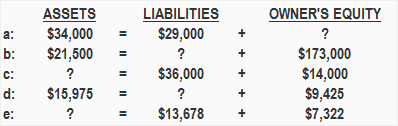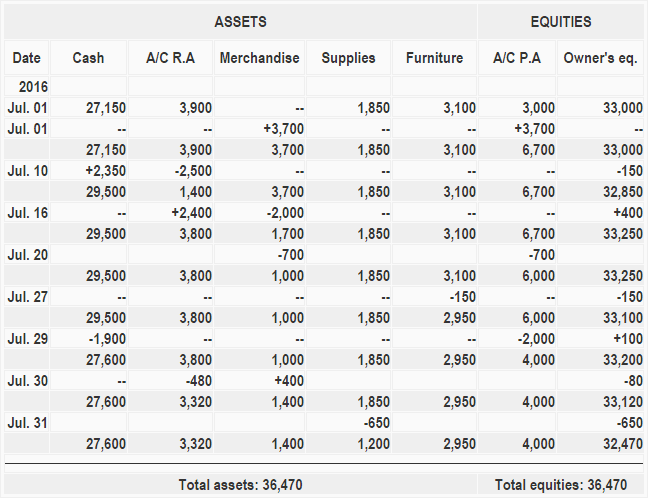The properties owned by a business are called assets. The rights or claims to the properties are referred to as equities.

For every business, the sum of the rights to the properties is equal to the sum of properties owned.

The relationship between the two may be expressed in the form of an equation. This equation is commonly known as the accounting equation and is written as follows:

Assets = Equities

In this equation, equities is separated into two parts:

1. The rights of creditors
2. The rights of owners

The rights of creditors represent debts of the business and are called liabilities. The rights of owners are called capital or owner’s equity. By substituting the two types of equities, we get the following well-known form of the accounting equation:

Assets = Liabilities + Owner’s equity

Creditors have preferential rights over the assets of the business, and so it is appropriate to place liabilities before the capital or owner’s equity in the equation.

If an item in the accounting equation is missing, we can easily compute it by solving the equation for that item. Consider the following example:

Example 1Required: Compute the missing figures in the above equations.

Solution

a: Owner’s equity = \$5,000 (\$34,000 – \$29,000)
b: Liabilities = \$4,200 (\$21,500 – \$17,300)
c: Assets = \$50,000 (\$36,000 + \$14,000)
d: Liabilities = \$6,550 (\$15,975 – \$9,425)
e: Assets = \$21,000 = (\$13,678 + \$7,322)

Effect of Transactions on the Accounting Equation

As transactions occur within a business, the amounts of assets, liabilities, and owner’s equity change. However, the overall equation always remains balanced.

The effects of changes in the items of the equation can be shown by the use of + or – signs placed against the affected items.

At this point, let’s consider another example and see how various transactions affect the amounts of the elements in the accounting equation.

Example 2

On 1 January 2016, Sam started a trading business called Sam Enterprises with an initial investment of \$100,000.

At this time, there is external equity or liability in Sam Enterprise. The only equity is Sam’s capital (i.e., owner’s equity amounting to \$100,000). The accounting equation at the start of the business would, therefore, look like the following:

On 2 January, Mr. Sam purchases a building for \$50,000 for use in the business. The impact of this transaction is a decrease in an asset (i.e., cash) and an addition of another asset (i.e., building).

After this transaction, the items of the equation would be affected as follows:

On 5 January, Sam purchases merchandise for \$20,000 on credit. As a result of the transaction, an asset in the form of merchandise increases, leading to an increase in the total assets.

On the other side of the equation, a liability (i.e., accounts payable) is created. The items of the accounting equation for Sam Enterprises would be affected as follows:

On 10 January, Sam Enterprises sells merchandise for \$10,000 cash and earns a profit of \$1,000. As a result of this transaction, an asset (i.e., cash) increases by \$10,000 while another asset ( i.e., merchandise) decreases by \$9,000 (the original cost).

This transaction also generates a profit of \$1,000 for Sam Enterprises, which would increase the owner’s equity element of the equation. The resulting equation would look appear as follows:

On 12 January, Sam Enterprises pays \$10,000 cash to its accounts payable. This transaction would reduce an asset (cash) and a liability (accounts payable). The equation items would be affected as follows:

On 18 January, merchandise costing \$5,000 is sold for \$6,000 on credit. It reduces an asset (merchandise) but also creates a new asset (accounts receivable) valued at \$6,000.

The difference between the sale price and the cost of merchandise is the profit of the business that would increase the owner’s equity by \$1,000 (6,000 – \$5,000). The accounting equation after this transaction would look like the following:

On 20 January, merchandise costing \$500 are stolen by a thief. The loss of goods by theft would reduce both merchandise and owner’s equity and change the equation elements as follows:

On 22 January, Sam Enterprises pays \$9,500 cash to creditors and receives a cash discount of \$500.

This transaction would reduce cash by \$9,500 and accounts payable by \$10,000. The difference of \$500 in the cash discount would be added to the owner’s equity.

On 25 January, a loan of \$5,000 is obtained from a bank. This transaction brings cash into the business and also creates a new liability called bank loan.

On 28 January, merchandise costing \$5,500 are destroyed by fire. The effect of this transaction on the accounting equation is the same as that of loss by fire that occurred on January 20.

The merchandise would decrease by \$5,500 and owner’s equity would also decrease by the same amount.

On 31 January, the electricity bill of \$500 is paid. This transaction would decrease cash and owner’s equity.

Example 3

The assets, liabilities, and owner’s equity of Modern Enterprises at the beginning of July 2016 are given below:

• Cash: \$27,150
• Accounts receivable: \$3,900
• Supplies: \$1,850
• Furniture: \$3,100
• Accounts payable: \$3,000
• Owners equity: \$33,000

The following transactions were performed during the month of July 2016:

• July 01: Merchandise purchased on account from Mr. A \$3,700
• July 10: Received cash from accounts receivable \$2,350 and allowed a discount of \$150
• July 16: Sold merchandise on account valued at \$2,400 to Mr. X; the cost of the merchandise was \$2,000
• July 20: Defective merchandise returned to Mr. A \$700
• July 27: Recorded depreciation on furniture \$150
• July 29: Paid cash to accounts payable \$1,900 and received a discount of \$100
• July 30: Merchandise returned by Mr. X \$480; the cost of the merchandise was \$400
• July 31: Cost of supply on hand at the end of July was \$1,200

Required: Show the effect of the above transactions on the accounting equation of Modern Enterprises.

SolutionTrue is a Certified Educator in Personal Finance (CEPF®), contributes to his financial education site, Finance Strategists, and has spoken to various financial communities such as the CFA Institute, as well as university students like his Alma mater, Biola University, where he received a bachelor of science in business and data analytics.

To learn more about True, visit his personal website, view his author profile on Amazon, his interview on CBS, or check out his speaker profile on the CFA Institute website.